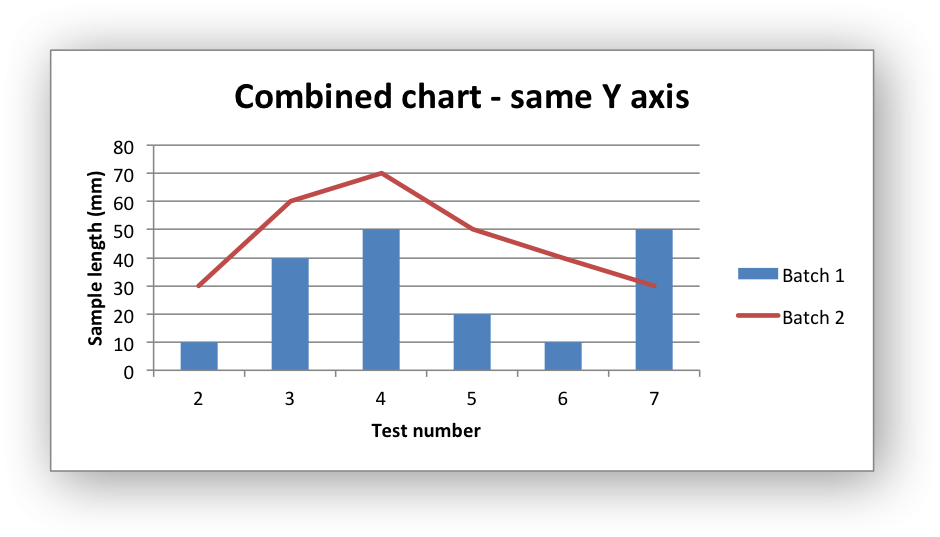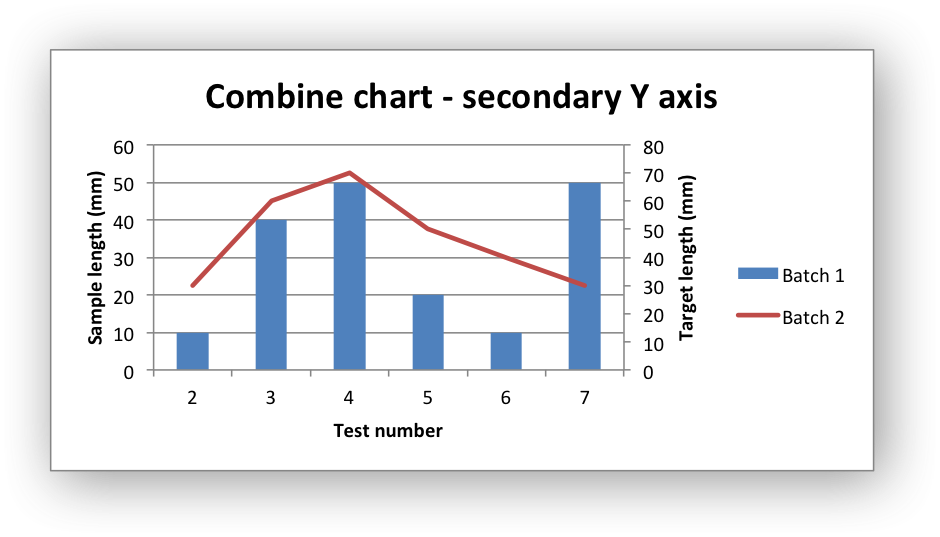# Example: Combined Chart

Example of creating combined Excel charts with two chart types.

In the first example we create a combined column and line chart that share the same X and Y axes.In the second example we create a similar combined column and line chart except that the secondary chart has a secondary Y axis.```#######################################################################
#
# An example of a Combined chart in XlsxWriter.
#
# Copyright 2013-2023, John McNamara, jmcnamara@cpan.org
#
from xlsxwriter.workbook import Workbook

workbook = Workbook("chart_combined.xlsx")

# Add the worksheet data that the charts will refer to.
headings = ["Number", "Batch 1", "Batch 2"]
data = [
[2, 3, 4, 5, 6, 7],
[10, 40, 50, 20, 10, 50],
[30, 60, 70, 50, 40, 30],
]

worksheet.write_column("A2", data)
worksheet.write_column("B2", data)
worksheet.write_column("C2", data)

#
# In the first example we will create a combined column and line chart.
# They will share the same X and Y axes.
#

# Create a new column chart. This will use this as the primary chart.

# Configure the data series for the primary chart.
{
"name": "=Sheet1!\$B\$1",
"categories": "=Sheet1!\$A\$2:\$A\$7",
"values": "=Sheet1!\$B\$2:\$B\$7",
}
)

# Create a new column chart. This will use this as the secondary chart.

# Configure the data series for the secondary chart.
{
"name": "=Sheet1!\$C\$1",
"categories": "=Sheet1!\$A\$2:\$A\$7",
"values": "=Sheet1!\$C\$2:\$C\$7",
}
)

# Combine the charts.
column_chart1.combine(line_chart1)

# Add a chart title and some axis labels. Note, this is done via the
# primary chart.
column_chart1.set_title({"name": "Combined chart - same Y axis"})
column_chart1.set_x_axis({"name": "Test number"})
column_chart1.set_y_axis({"name": "Sample length (mm)"})

# Insert the chart into the worksheet
worksheet.insert_chart("E2", column_chart1)

#
# In the second example we will create a similar combined column and line
# chart except that the secondary chart will have a secondary Y axis.
#

# Create a new column chart. This will use this as the primary chart.

# Configure the data series for the primary chart.
{
"name": "=Sheet1!\$B\$1",
"categories": "=Sheet1!\$A\$2:\$A\$7",
"values": "=Sheet1!\$B\$2:\$B\$7",
}
)

# Create a new column chart. This will use this as the secondary chart.

# Configure the data series for the secondary chart. We also set a
# secondary Y axis via (y2_axis). This is the only difference between
# this and the first example, apart from the axis label below.
{
"name": "=Sheet1!\$C\$1",
"categories": "=Sheet1!\$A\$2:\$A\$7",
"values": "=Sheet1!\$C\$2:\$C\$7",
"y2_axis": True,
}
)

# Combine the charts.
column_chart2.combine(line_chart2)

# Add a chart title and some axis labels.
column_chart2.set_title({"name": "Combine chart - secondary Y axis"})
column_chart2.set_x_axis({"name": "Test number"})
column_chart2.set_y_axis({"name": "Sample length (mm)"})

# Note: the y2 properties are on the secondary chart.
line_chart2.set_y2_axis({"name": "Target length (mm)"})

# Insert the chart into the worksheet
worksheet.insert_chart("E18", column_chart2)

workbook.close()
```